### Example 63.4 Affecting Curvature through Parameterization

The work of Ratkowsky (1983, 1990) has brought into focus the importance of close-to-linear behavior of parameters in nonlinear regression models. The curvature in a nonlinear model consists of two components: the intrinsic curvature and the parameter-effects curvature. See the section Relative Curvature Measures of Nonlinearity for details. Intrinsic curvature expresses the degree to which the nonlinear model bends as values of the parameters change. This is not the same as the curviness of the model as a function of the covariates (the x variables). Intrinsic curvature is a function of the type of model you are fitting and the data. This curvature component cannot be affected by reparameterization of the model. According to Ratkowsky (1983), the intrinsic curvature component is typically smaller than the parameter-effects curvature, which can be affected by altering the parameterization of the model.

In models with low curvature, the nonlinear least squares parameter estimators behave similarly to least squares estimators in linear regression models, which have a number of desirable properties. If the model is correct, they are best linear unbiased estimators and are normally distributed if the model errors are normal (otherwise they are asymptotically normal). As you lower the curvature of a nonlinear model, you can expect that the parameter estimators approach the behavior of the linear regression model estimators: they behave close to linear.

This example uses a simple data set and a commonly applied model for dose-response relationships to examine how the parameter-effects curvature can be reduced. The statistics by which an estimator’s behavior is judged are Box’s bias (Box, 1971) and Hougaard’s measure of skewness (Hougaard, 1982, 1985).

The log-logistic model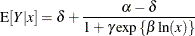is a popular model to express the response Y as a function of dose x. The response is bounded between the asymptotesand
. The term in the denominator governs the transition between the asymptotes and depends on two parameters,and. The log-logistic model can be viewed as a member of a broader class of dose-response functions, those relying on switch-on or switch-off mechanisms (see, for example, Schabenberger and Pierce 2002, sec. 5.8.6). A switch function is usually a monotonic function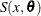that takes values between 0 and 1. A switch-on function increases in x; a switch-off function decreases in x. In the log-logistic case, the function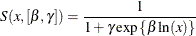is a switch-off function for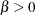and a switch-on function for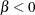. You can write general dose-response functions with asymptotes simply as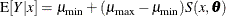The following DATA step creates a small data set from a dose-response experiment with response y:

data logistic;
input dose y;
logdose = log(dose);
datalines;
0.009  106.56
0.035   94.12
0.07    89.76
0.15    60.21
0.20    39.95
0.28    21.88
0.50     7.46
;


A graph of these data is produced with the following statements:

proc sgplot data=logistic;
scatter y=y x=dose;
xaxis type=log logstyle=linear;
run;


Output 63.4.1: Observed Data in Dose-Response Experiment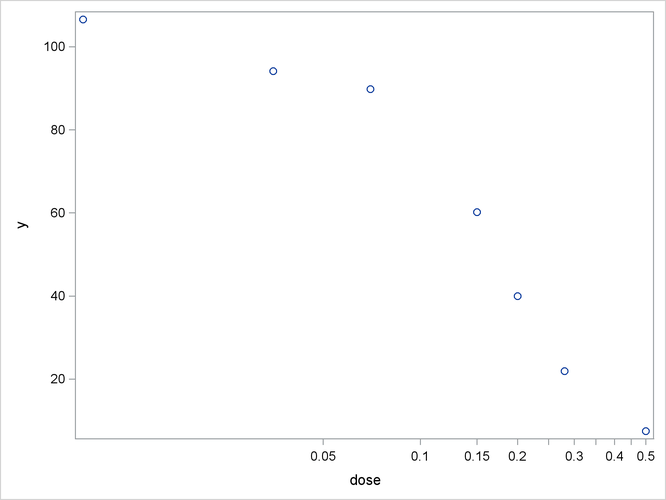When dose is expressed on the log scale, the sigmoidal shape of the dose-response relationship is clearly visible (Output 63.4.1). The log-logistic switching model in the preceding parameterization is fit with the following statements in the NLIN procedure:

proc nlin data=logistic bias hougaard nlinmeasures;
parameters alpha=100 beta=3 gamma=300;
delta = 0;
Switch = 1/(1+gamma*exp(beta*log(dose)));
model y = delta + (alpha - delta)*Switch;
run;


The lower asymptoteis assumed to be 0 in this case. Sinceis not listed in the PARAMETERS statement and is assigned a value in the program, it is assumed to be constant. Note that the term Switch is the switch-off function in the log-logistic model. The BIAS and HOUGAARD options in the PROC NLIN statement request that Box’s bias, percentage bias, and Hougaard’s skewness measure be added to the table of parameter estimates, and the NLINMEASURES option requests that the global nonlinearity measures be produced.

The NLIN procedure converges after 10 iterations and achieves a residual mean squared error of 15.1869 (Output 63.4.2). This value is not that important by itself, but it is worth noting since this model fit is compared to the fit with other parameterizations later on.

Output 63.4.2: Iteration History and Analysis of Variance

The NLIN Procedure
Dependent Variable y
Method: Gauss-Newton

Iterative Phase
Iter alpha beta gamma Sum of Squares
0 100.0 3.0000 300.0 386.4
1 100.4 2.8011 162.8 129.1
2 100.8 2.6184 101.4 69.2710
3 101.3 2.4266 69.7579 68.2167
4 101.7 2.3790 69.0358 60.8223
5 101.8 2.3621 67.3709 60.7516
6 101.8 2.3582 67.0044 60.7477
7 101.8 2.3573 66.9150 60.7475
8 101.8 2.3571 66.8948 60.7475
9 101.8 2.3570 66.8902 60.7475
10 101.8 2.3570 66.8892 60.7475

 NOTE: Convergence criterion met.

 Note: An intercept was not specified for this model.

Source DF Sum of Squares Mean Square F Value Approx
Pr > F
Model 3 33965.4 11321.8 745.50 <.0001
Error 4 60.7475 15.1869
Uncorrected Total 7 34026.1

The table of parameter estimates displays the estimates of the three model parameters, their approximate standard errors, 95% confidence limits, Hougaard’s skewness measure, Box’s bias, and percentage bias (Output 63.4.3). Parameters for which the skewness measure is less than 0.1 in absolute value and with percentage bias less than 1% exhibit very close-to-linear behavior, and skewness values less than 0.25 in absolute value indicate reasonably close-to-linear behavior (Ratkowsky, 1990). According to these rules, the estimators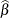and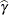suffer from substantial curvature. The estimatoris especially far-from-linear. Inferences that involveand rely on the reported standard errors or confidence limits (or both) for this parameter might be questionable.

Output 63.4.3: Parameter Estimates, Hougaard’s Skewness, and Box’s Bias

Parameter Estimate Approx
Std Error
Approximate 95% Confidence
Limits
Skewness Bias Percent
Bias
alpha 101.8 3.0034 93.4751 110.2 0.1415 0.1512 0.15
beta 2.3570 0.2928 1.5440 3.1699 0.4987 0.0303 1.29
gamma 66.8892 31.6146 -20.8870 154.7 1.9200 10.9230 16.3

The related global nonlinearity measures output table (Output 63.4.4) shows that both the maximum and RMS parameter-effects curvature are substantially larger than the critical curvature value recommended by Bates and Watts (1980). In contrast, the intrinsic curvatures of the model are less than the critical value. This implies that most of the nonlinearity can be removed by reparameterization.

Output 63.4.4: Global Nonlinearity Measures

Global Nonlinearity Measures
Max Intrinsic Curvature 0.2397
RMS Intrinsic Curvature 0.1154
Max Parameter-Effects Curvature 4.0842
RMS Parameter-Effects Curvature 1.8198
Curvature Critical Value 0.3895
Raw Residual Variance 15.187
Projected Residual Variance 5.922

One method of reducing the parameter-effects curvature, and thereby reduce the bias and skewness of the parameter estimators, is to replace a parameter with its expected-value parameterization. Schabenberger et al. (1999) and Schabenberger and Pierce (2002, sec. 5.7.2) refer to this method as reparameterization through defining relationships. A defining relationship is obtained by equating the mean response at a chosen value of x (say,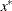) to the model: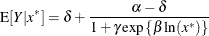This equation is then solved for a parameter that is subsequently replaced in the original equation. This method is particularly useful ifhas an interesting interpretation. For example, let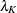denote the value that reduces the response by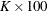%,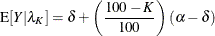Becauseexhibits large bias and skewness, it is the target in the first round of reparameterization. Setting the expression for the conditional mean atequal to the mean function when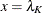yields the following expression: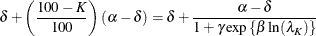This expression is solved for, and the result is substituted back into the model equation. This leads to a log-logistic model in whichis replaced by the parameter, the dose at which the response was reduced by%. The new model equation is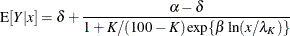A particularly interesting choice is K = 50, since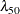is the dose at which the response is halved. In studies of mortality, this concentration is also known as the LD50. For the special case ofthe model equation becomes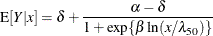You can fit the model in the LD50 parameterization with the following statements:

proc nlin data=logistic bias hougaard;
parameters alpha=100 beta=3 LD50=0.15;
delta = 0;
Switch = 1/(1+exp(beta*log(dose/LD50)));
model y = delta + (alpha - delta)*Switch;
output out=nlinout pred=p lcl=lcl ucl=ucl;
run;


Partial results from this NLIN run are shown in Output 63.4.5. The analysis of variance tables in Output 63.4.2 and Output 63.4.5 are identical. Changing the parameterization of a model does not affect the model fit. It does, however, affect the interpretation of the parameters and the statistical properties (close-to-linear behavior) of the parameter estimators. The skewness and bias measures of the parameter LD50 is considerably reduced compared to those values for the parameterin the previous parameterization. Also,has been replaced by a parameter with a useful interpretation, the dose that yields a 50% reduction in mean response. Also notice that the bias and skewness measures ofandare not affected by the
LD50 reparameterization.

Output 63.4.5: ANOVA Table and Parameter Estimates in LD50 Parameterization

The NLIN Procedure
Dependent Variable y
Method: Gauss-Newton

 NOTE: Convergence criterion met.

 Note: An intercept was not specified for this model.

Source DF Sum of Squares Mean Square F Value Approx
Pr > F
Model 3 33965.4 11321.8 745.50 <.0001
Error 4 60.7475 15.1869
Uncorrected Total 7 34026.1

Parameter Estimate Approx
Std Error
Approximate 95% Confidence
Limits
Skewness Bias Percent
Bias
alpha 101.8 3.0034 93.4752 110.2 0.1415 0.1512 0.15
beta 2.3570 0.2928 1.5440 3.1699 0.4987 0.0303 1.29
LD50 0.1681 0.00915 0.1427 0.1935 -0.0605 -0.00013 -0.08

To reduce the parameter-effects curvature of theparameter, you can use the technique of defining relationships again. This can be done generically, by solving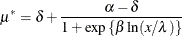for, treating
as the new parameter (in lieu of), and choosing a value forthat leads to low skewness. This results in the expected-value parameterization of. Solving foryields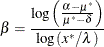The interpretation of the parameterthat replacesin the model equation is simple: it is the mean dose response when the dose is. Fixing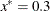, the following PROC NLIN statements fit this model:

proc nlin data=logistic bias hougaard nlinmeasures;
parameters alpha=100 mustar=20 LD50=0.15;
delta   = 0;
xstar   = 0.3;
beta    = log((alpha - mustar)/(mustar - delta)) / log(xstar/LD50);
Switch  = 1/(1+exp(beta*log(dose/LD50)));
model y = delta + (alpha - delta)*Switch;
output out=nlinout pred=p lcl=lcl ucl=ucl;
run;


Note that the switch-off function continues to be written in terms ofand the LD50. The only difference from the previous model is thatis now expressed as a function of the parameter. Using expected-value parameterizations is a simple mechanism to lower the curvature in a model and to arrive at starting values. The starting value forcan be gleaned from Output 63.4.1 at x = 0.3.

Output 63.4.6 shows selected results from this NLIN run. The ANOVA table is again unaffected by the change in parameterization. The skewness foris significantly reduced in comparison to those of theparameter in the previous model (compare Output 63.4.6 and Output 63.4.5), while its bias remains on the same scale from Output 63.4.5 to Output 63.4.6. Also note the substantial reduction in the parameter-effects curvature values. As expected, the intrinsic curvature values remain intact.

Output 63.4.6: ANOVA Table and Parameter Estimates in Expected-Value Parameterization

The NLIN Procedure
Dependent Variable y
Method: Gauss-Newton

 NOTE: Convergence criterion met.

 Note: An intercept was not specified for this model.

Source DF Sum of Squares Mean Square F Value Approx
Pr > F
Model 3 33965.4 11321.8 745.50 <.0001
Error 4 60.7475 15.1869
Uncorrected Total 7 34026.1

Parameter Estimate Approx
Std Error
Approximate 95% Confidence
Limits
Skewness Bias Percent
Bias
alpha 101.8 3.0034 93.4752 110.2 0.1415 0.1512 0.15
mustar 20.7073 2.6430 13.3693 28.0454 -0.0572 -0.0983 -0.47
LD50 0.1681 0.00915 0.1427 0.1935 -0.0605 -0.00013 -0.08

Global Nonlinearity Measures
Max Intrinsic Curvature 0.2397
RMS Intrinsic Curvature 0.1154
Max Parameter-Effects Curvature 0.2925
RMS Parameter-Effects Curvature 0.1500
Curvature Critical Value 0.3895
Raw Residual Variance 15.187
Projected Residual Variance 5.9219